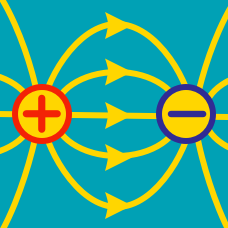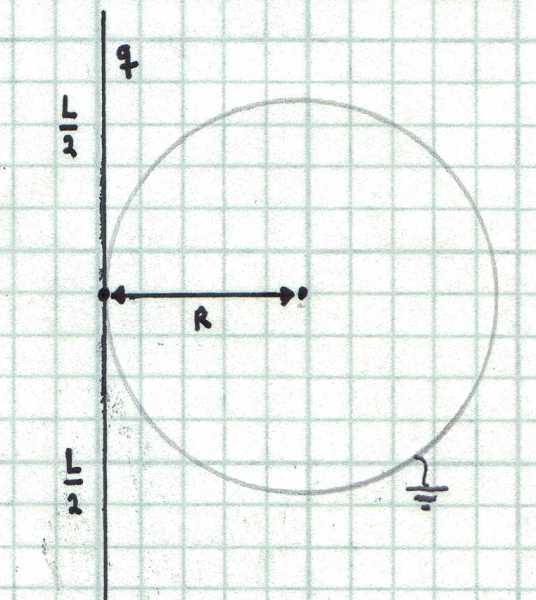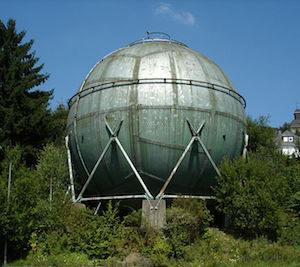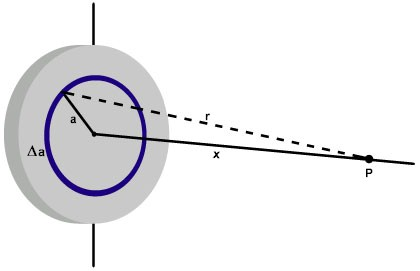Electricity and Magnetism

# Electric Fields: Level 4-5 Challenges

Find the electrostatic force of interaction between two parallel non conducting wires each of length $l$ , charge density per unit length $\lambda$, and separated by a distance $d$.

Give your answer as the value of this force in $\text{ Newtons }$ using $\lambda = 10 \mu \text{C/ m}$, $l = 1 \text{m}$, $\text{ d = 1m }, \frac{1}{4 \pi \epsilon_{0} } = 9 \times 10^9\text{ N } m^2/ C^2$

Note:

The wires completely face each other (like if their equations were $0 \leq x \leq L, y,z= 0$ and $0 \leq x \leq L, y= d, z=0$)Consider a dielectric thin rod with total charge $q=1~\mu\text{C}$ uniformly distributed over its length. The rod touches a grounded conducting sphere of radius $R=50~\text{cm}$ as shown in the figure below. Find the charged induced in the sphere in microcoulombs if the length of the rod is $L=1~\text{m}$. The following integral may be useful: $\int \frac{1}{\sqrt{a^{2}+x^{2}}}dx=\ln(x+\sqrt{x^2+a^{2}})+C.$

Three identical point charges, each with mass $m=1~\text{g}$, are connected by strings of the same length $l$ so that they form an equilateral triangle ABC. Initially, the charges are at rest and the electrostatic potential energy of the system is $U_{0}=1~\text{J}$. Suddenly, the string connecting the charges at A and B snaps. What will be the maximum speed in meters per second of the charge located at C.An earthed metallic sphere is kept in a uniform electric field $E_0 \hat{k}$. If $V(r, \theta ,\phi )$ denotes the potential function for the region outside the sphere then the value of $\displaystyle \frac{V \left( 2R, \frac{\pi }{3} , \frac{ \pi }{2} \right) }{ V \left( 3R, \frac{-\pi }{3} , \frac{ 2\pi }{3} \right) }$ can be expressed as $\dfrac{a}{b}$ for some positive coprime integers $a,b$.

Evaluate $a+b$.

Details and Assumptions:

• $\hat{k}$ is the unit vector along the direction of $z$ axis in a normal right handed Cartesian coordinate system.

• Reference potential i.e. $(V=0)$ is obviously the sphere since it is grounded.

• $R$ is the radius of the sphere.

###### Image credit: Wikipedia Bob lonescuA particle $P$ having a charge of $-1\ \mu\text{C}$ and mass 2 g is held at a distance of $x=1\text{ m}$ from the center of a disc, as shown in the figure above. The disc has a radius of 2 m and surface charge density $6.84\times 10^{-4} \text{ C/m}^2$.

The particle is released, and flies toward the disc. Find the velocity (in m/s) of the particle when it has covered half the distance.

×